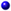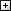Processing ......FreeComputerBooks.com Free Computer, Mathematics, Technical Books and Lecture Notes, etc.

Mathematical Methods for Economic Theory
How many flights will depart from a particular airport? Click here to find out.
• Title Mathematical Methods for Economic Theory
• Author(s) Martin J. Osborne
• Publisher: University of Toronto
• Hardcover/Paperback: N/A
• ebook HTML
• Language: English
• ISBN-10: N/A
• ISBN-13: N/ABook Description

This book provides an understanding of the mathematical propositions necessary for comprehensively following the fundamentals of economic theory. These mathematical tools help the student to define terms precisely and apply them to real world situations and also to the needs of economics like problems of decision making, inflation, forecast, and risk.

It covers the basic mathematical tools used in economic theory. Knowledge of elementary calculus is assumed; some of the prerequisite material is reviewed in the first section. The main topics are multivariate calculus, concavity and convexity, optimization theory, differential equations, and difference equations.

It emphasizes techniques rather than abstract theory. However, the conditions under which each technique is applicable are stated precisely.

Reviews, Ratings, and Recommendations: Related Books and Categories: Read and Download Links:Similar Books:All CategoriesRecent BooksIT Research LibraryMiscellaneous BooksComputer LanguagesComputer ScienceData Science/DatabasesElectronic EngineeringJava and Java EE (J2EE)Linux and UnixMathematicsMicrosoft and .NETMobile ComputingNetworking and CommunicationsSoftware EngineeringSpecial TopicsWeb Programming